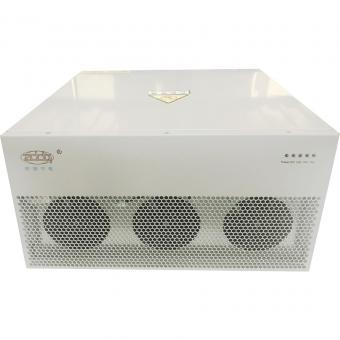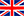English

###### Fundamental components of Harmonics and filters
Nov 23,2019

Fundamental (component)

The component of order 1 of the Fourier series of a periodic quantity. (IEV 161-02-17)

Harmonic

The component of the Fourier-series decomposition of a volt- age or current periodic wave. (IEV 161-02-18 modified)

Harmonic order (h)

The ratio of the frequency of a harmonic (fh) to the fundamen- tal (rated) network frequency (f1). (IEV 161-02-19 modified)

Odd harmonics

Harmonics with odd harmonic order.

Even harmonics

Harmonics with even harmonic order.

Characteristic harmonics

Those harmonics produced by static converters during theo- retically ideal operation. The characteristic harmonic order of static AC/DC converters is given by h = mp ± 1, where p is the pulse number of the converter and m is any integer.

For example, the six-pulse converter circuit has characteristic harmonics with order numbers h = 5, 7, 11, 13,1 7, 19... .(IEC 61642)

Non-characteristic harmonics

Those harmonics that are produced as a result of imbalance in the AC power system or asymmetrical delay of the fir-

ing angle of the converter. They are also produced by other non-linear, time-varying devices, for example frequency converters, fluorescent lamps, arc furnaces, electric welding machines, etc. (IEC 61642)

Filter

A device generally constituted by reactors, capacitors and re- sistors if required, tuned to present a known impedance over a given frequency range. (IEC 61642)

Tuning frequency

The frequency at which the filter impedance, calculated from the rated values, has a minimum or maximum value.

(IEC 61642)

Tuned filter

A filter with a tuning frequency that differs by no more than 10% from the frequency that is to be filtered. (IEC 61642)

Detuned filter

A filter with a tuning frequency more than 10% below the lowest harmonic frequency with considerable current/voltage amplitude. (IEC 61642)

Damped filter

A filter with low, predominantly resistive, impedance over a wide band of frequencies. (IEC 61642)

Band-pass filter

A reactor (inductance) connected in series with a capacitor (capacitance).

High-pass filter

A damped band-pass filter. A resistor is connected in parallel with the reactor in a band-pass filter, which creates damping on the parallel resonance.

C-type filter

High-pass filter modified with an extra capacitor to short- circuit the resistor for the fundamental frequency. Alternative when a damped filter is needed for a low-tuned filter (normally n < 5).

Double-tuned filter

Filter configuration with two tuning frequencies, mainly used in HVDC filter systems.

Displacement factor

The ratio of the active power of the fundamental wave to the apparent power of the fundamental wave.(IEV 131-03-21 modified)

Distortion factor

The ratio of the rms value of the harmonic content to the rms value of the fundamental quantity, expressed as a percentage of the fundamental frequency. (IEV 131-03-04 modified)

DF = (sum of the squares of rms values of the harmonics)1/2 / rms value of the fundamental frequency x 100%

Total Harmonic Distortion, THD

The square root of the sum of squares of all individual har- monic distortions (voltages or currents) is called the Total Harmonic Distortion (THD).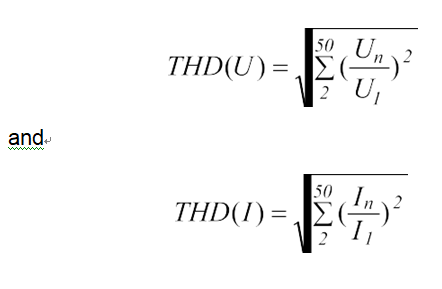Un = harmonic voltage

U1 = fundamental voltage

In = harmonic voltage

I1 = fundamental voltage

Blog
Hot Products
•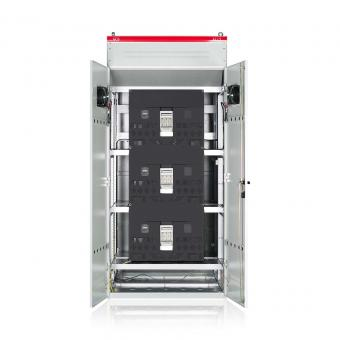#### Capacitor banks and harmonic filters. Low voltage

•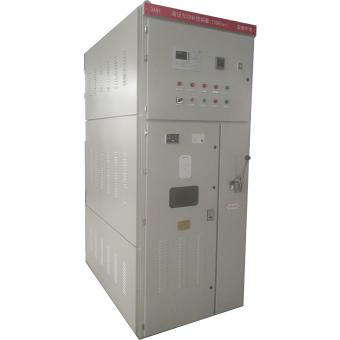#### 3.3KV Local reactive power compensation for motors,Arc Furnace

•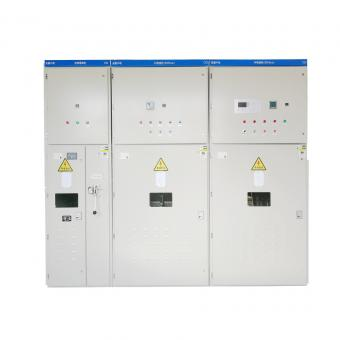#### Medium voltage Automatic Capacitor banks with harmonic filters

•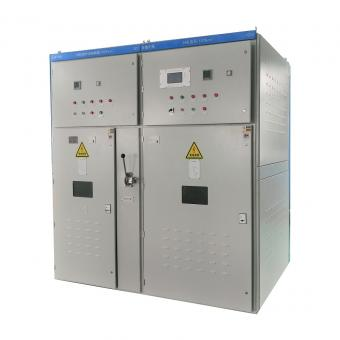#### High voltage Automatic Power Factor improvement plant for Motors VFDs

•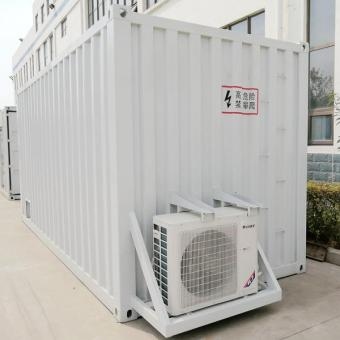#### High voltage static var generator HV SVG Statcom outdoor container

•#### Medium voltage outdoor Static Synchronous Compensator(STATCOM)

•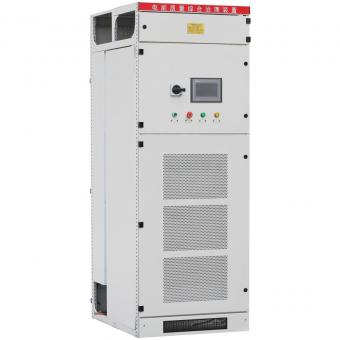•